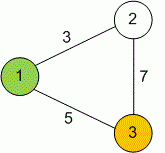Competitions

# Maximum flow

Find the maximum flow in the given network.

#### Input

The first line contains two integers n and m (1n100, 1m10000) - respectively the number of vertices and edges in the network. Each of the following m lines contains three numbers ui, vi and ci (1ui, vin, 1ci10000), meaning that between vertices ui and vi in the network there is an edge with capacity ci. Vertex 1 is the source, and the vertex n is the destination. Graph of the network is undirected and can contain multiple edges. All numbers are integers.

#### Output

Print the maximum flow in the given network.Time limit 1 second
Memory limit 128 MiB
Input example #1
3 3
1 2 3
1 3 5
3 2 7

Output example #1
8